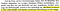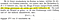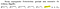# How Georg Cantor came to take the first step beyond infinity

A few weeks ago, I received a book with the collected writings of Georg Cantor, edited by Ernst Zermelo and entitled “Georg Cantor: Gesammelte Abhandlungen: Mathematischen und Philosophischen Inhalts.” It is a reprint of the original from 1932. I had read some Cantor before, but having all of his writing in one handy tome, with some comments by Zermelo, was really inspiring. Hence this article.

Warning: some knowledge of the German language will be useful, also I have not used mathematical formulas because of Medium’s limitations.

# A first encounter with accumulation points (1870)

It is interesting to note that he took care to actually define convergence to zero:

He then proceeded to meticulously prove what, in today’s mathematical language, would simply be: “any bounded set of real numbers with a single accumulation point at 0 converges to 0.” These concepts seemed to be relatively new at the time.

# Derived sets: the seed of set theory is planted (1872)

1. Cantor presents a novel construction of real numbers, based on Cauchy sequences;
2. while doing this, he introduces the concept of extending an “Area” A into an Area B by using infinite sequences, and then successively to Areas C, D etc. He notes that such a process “.. carries in it the seed of a necessary and absolutely infinite extension”
3. he then defines accumulation points [link] and derived sets [link] of sets of real numbers. A derived set consists of the accumulation points of a given set, and starting with a set P this derivation can be applied successively, resulting in derived sets P’, P’’, P’’’ etc.The extension of Areas from A to B to C etc. “.. carries in it the seed of a necessary and absolutely infinite extension”

The discussion of these 3 points represents over two thirds of his paper. Cantor then proceeds to use this new concept of derived sets to prove a not exactly earth-shattering theorem about trigonometric series.

Interestingly, while Cantor explicitly mentions that the process of generating one Area from another might proceed to infinity, he refrains from doing so for derived sets. And yet it is clear that applying that single idea, of extending the sequence of derived sets to infinity, would later naturally and almost obviously lead to the theory of transfinite ordinals. That is why Zermelo sees this paper, and especially its section on derived sets, as the actual Seed of set theory — cf a note in Cantor’s collected works, mentioned above.

# Infinity plus one (1880)

First, a remark about the empty set, which we take for granted today, and on which the entire edifice of modern mathematics rests. In his 1872 paper, introducing derived sets, Cantor defines a set of points as being of the n-th form if its n-th derivation has a finite number of points, and therefore has no derived set. Would it not have been more natural to define a set as being of the n-th type if its n-th derivation is the empty set? The answer is actually no, but the initial reason might have been that the concept of an empty set was not yet developed. It is only in his 1880 paper that Cantor introduces the empty set, denoted “0”.Cantor defines the empty set, noting that it is strictly speaking not a set.

On the very next page, the leap to infinity is taken. Probably for the first time in the history of mathematics, the result of an iteration over ALL natural numbers ends in a state which is not final, as it would be for a converging series, but from which additional steps can be taken. After infinity, there in infinity plus one, and it is different from infinity.

And if that was not enough, just a few lines later Cantor introduces notations that must, at least initially, have looked like utter madness to any mathematician of the time:infinity to the power of infinity to the power of infinity

# The first step beyond infinity, visualised

Let’s start with a simple example of a set S2 constituted by a series of points that converge to 0. The derived set of S2 is S1, constituted of the single point 0. The derived set of S1 is the empty set.

For each point s in S2, we can define a series of points that converges to s. We do this so that there is no overlap between these series, which are shown as S3 below. Then, for each of the points of S3, we can again define a non-overlapping series — this is shown as S4 in the following chart. Each of these series, indicated by red vertical lines, has a (countably) infinite number of points, and starting with S3 there is a (countably) infinite number of such series. Again, note that none of these series overlap.

It is clear that we can thus construct an infinite sequence S1, S2, … Sn … of sets of points, with Sn yielding the empty set after exactly n derivations.

To obtain the desired result, we start by dividing some interval into an infinite series of non-overlapping intervals. The next chart shows what happens when we “squeeze” S2 into the first of these intervals, S3 into the second, etc. The fact that we start with S2 is arbitrary, the chart just looks nicer that way. Let’s call the set resulting from this infinite union SI. Imagine all of these points projected on one vertical line.

We see that if we take any finite number m, the m-th derived set will always have an infinite number of elements, will be ever closer to 0 as m grows, and will always include 0. In other words, taking the limit of derived sets, with m tending to infinity, we obtain the set {0}. This can be denoted SI(∞) = {0}.

But now, after having completed an infinite number of derivation steps and being left with {0}, we can take exactly one extra derivation step to end the process: SI(∞)’ = {0}’ = ∅.

SI(∞)’ is denoted SI(∞+1) and it is clearly different from SI(∞) in our example. Later on, starting in 1883, Cantor will use ω instead of ∞ to denote this first infinity.